# `sklearn.model_selection`.train_test_split¶

`sklearn.model_selection.``train_test_split`(*arrays, **options)[source]

Split arrays or matrices into random train and test subsets

Quick utility that wraps input validation and `next(ShuffleSplit().split(X, y))` and application to input data into a single call for splitting (and optionally subsampling) data in a oneliner.

Read more in the User Guide.

Parameters: *arrays : sequence of indexables with same length / shape Allowed inputs are lists, numpy arrays, scipy-sparse matrices or pandas dataframes. test_size : float, int, None, optional If float, should be between 0.0 and 1.0 and represent the proportion of the dataset to include in the test split. If int, represents the absolute number of test samples. If None, the value is set to the complement of the train size. By default, the value is set to 0.25. The default will change in version 0.21. It will remain 0.25 only if `train_size` is unspecified, otherwise it will complement the specified `train_size`. train_size : float, int, or None, default None If float, should be between 0.0 and 1.0 and represent the proportion of the dataset to include in the train split. If int, represents the absolute number of train samples. If None, the value is automatically set to the complement of the test size. random_state : int, RandomState instance or None, optional (default=None) If int, random_state is the seed used by the random number generator; If RandomState instance, random_state is the random number generator; If None, the random number generator is the RandomState instance used by np.random. shuffle : boolean, optional (default=True) Whether or not to shuffle the data before splitting. If shuffle=False then stratify must be None. stratify : array-like or None (default is None) If not None, data is split in a stratified fashion, using this as the class labels. splitting : list, length=2 * len(arrays) List containing train-test split of inputs. New in version 0.16: If the input is sparse, the output will be a `scipy.sparse.csr_matrix`. Else, output type is the same as the input type.

Examples

```>>> import numpy as np
>>> from sklearn.model_selection import train_test_split
>>> X, y = np.arange(10).reshape((5, 2)), range(5)
>>> X
array([[0, 1],
[2, 3],
[4, 5],
[6, 7],
[8, 9]])
>>> list(y)
[0, 1, 2, 3, 4]
```
```>>> X_train, X_test, y_train, y_test = train_test_split(
...     X, y, test_size=0.33, random_state=42)
...
>>> X_train
array([[4, 5],
[0, 1],
[6, 7]])
>>> y_train
[2, 0, 3]
>>> X_test
array([[2, 3],
[8, 9]])
>>> y_test
[1, 4]
```
```>>> train_test_split(y, shuffle=False)
[[0, 1, 2], [3, 4]]
```

## Examples using `sklearn.model_selection.train_test_split`¶Faces recognition example using eigenfaces and SVMs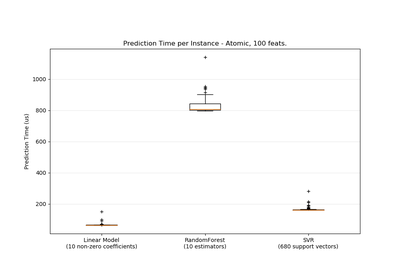Prediction Latency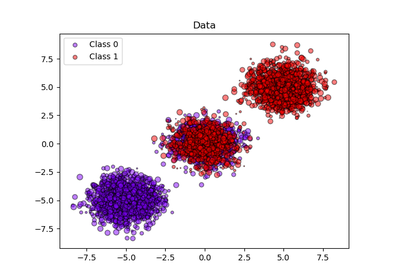Probability calibration of classifiers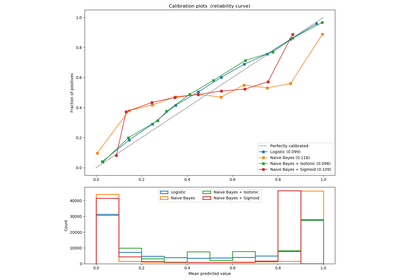Probability Calibration curves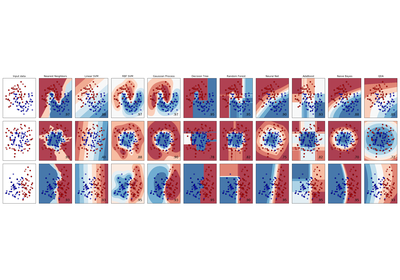Classifier comparison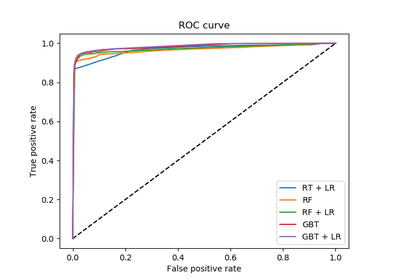Feature transformations with ensembles of trees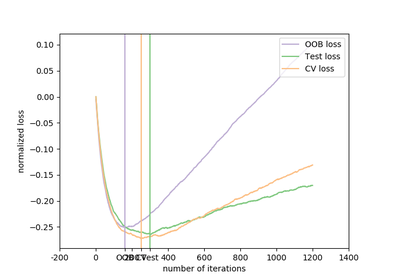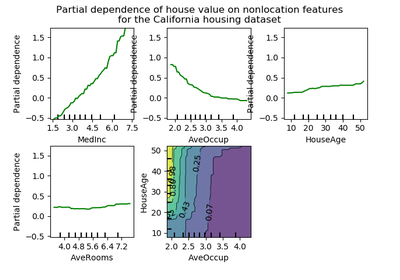Partial Dependence Plots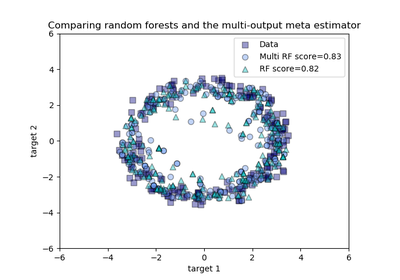Comparing random forests and the multi-output meta estimatorPipeline Anova SVM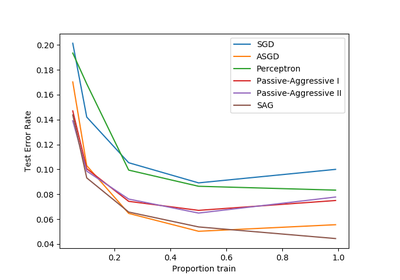Comparing various online solvers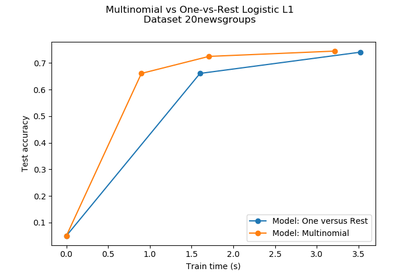Multiclass sparse logisitic regression on newgroups20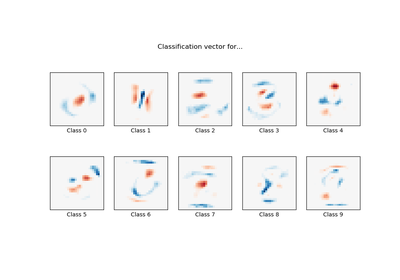MNIST classfification using multinomial logistic + L1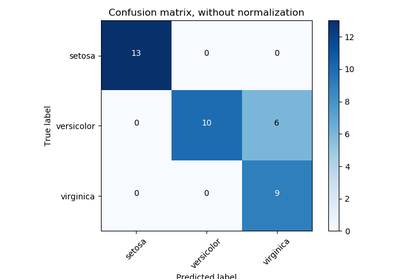Confusion matrixParameter estimation using grid search with cross-validation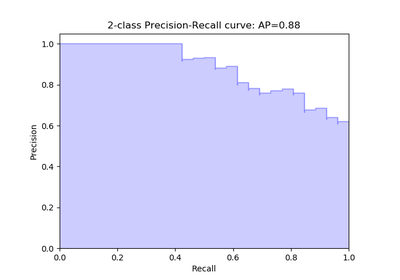Precision-Recall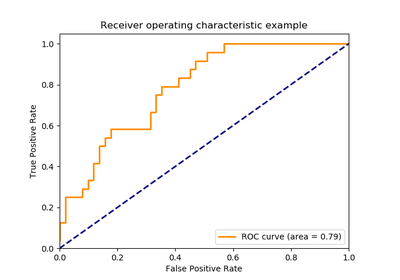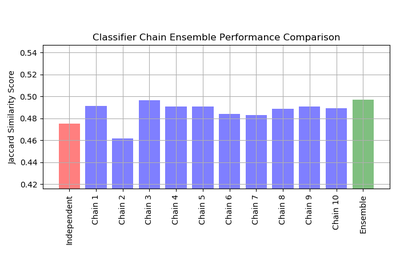Classifier Chain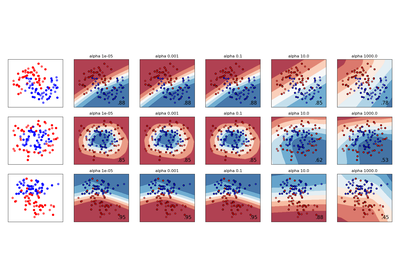Varying regularization in Multi-layer Perceptron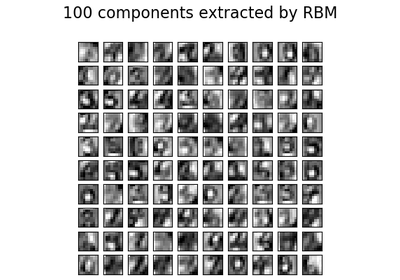Restricted Boltzmann Machine features for digit classification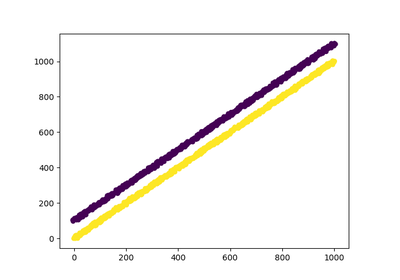Using FunctionTransformer to select columns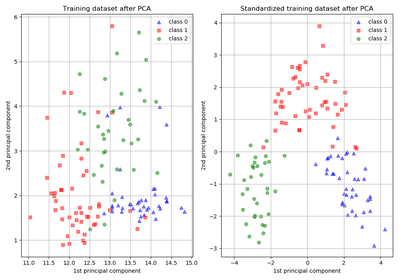Importance of Feature ScalingUnderstanding the decision tree structure